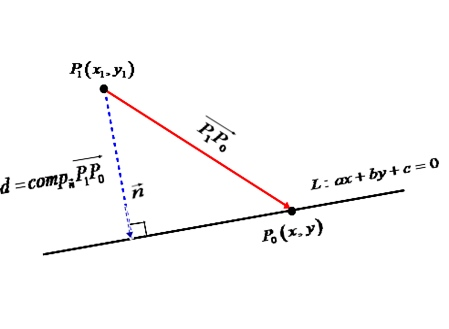# Use a scalar projection to show that the distance from a point P_1(x_1, y_1) to the line ax + by...

## Question:

Use a scalar projection to show that the distance from a point {eq}P_1(x_1, y_1) {/eq} to the line {eq}ax + by + c= 0 {/eq} is

{eq}\displaystyle \frac {|ax_1 + by_1 + c|}{ \sqrt {a^2 + b^2} } {/eq}

## Distance Between a Point and a Line and Scalar Projection:

When we know the direction vector of a line and a point {eq}{P_1}\left( {{x_1},{y_1}} \right) {/eq} outside the line we can set a normal vector {eq}{\vec n} {/eq} to the line that goes from point {eq}{P_1} {/eq} outside the line. Then we can calculate the scalar projection of any vector that goes from point {eq}{P_1} {/eq} to any point {eq}{P_0}\left( {x,y} \right) {/eq} on the line onto the normal vector {eq}{\vec n} {/eq}, so we get the distance between {eq}{P_1} {/eq} and the line: {eq}\,d = \,com{p_{\vec n}}\overrightarrow {{P_1}{P_0}} = \frac{{\vec n \cdot \overrightarrow {{P_1}{P_0}} }}{{\left| {\vec n} \right|}} {/eq}.

{eq}\eqalign{ & {\text{We have that the equation of the line is }}\,ax + by + c = 0.{\text{ Then its direction vector }} \cr & {\text{(vector parallel to the line) is }}\vec v = \left\langle {b, - a} \right\rangle \cr & {\text{So}}{\text{, two vector }}{\mkern 1mu} \vec v = \left\langle {{v_x},{v_y}} \right\rangle {\mkern 1mu} {\text{ and }}{\mkern 1mu} \vec n{\text{ are perpendicular if }}{\mkern 1mu} \vec n = \left\langle {{v_y}, - {v_x}} \right\rangle {\text{, thus the normal}} \cr & {\text{vector to the line is: }}\,\vec n = \left\langle {a,b} \right\rangle {\text{.}} \cr & {\text{Now we establish a vector between the point }}\,{P_1}\left( {{x_1},{y_1}} \right){\text{ outside the line and a }} \cr & {\text{point }}\,{P_0}\left( {x,y} \right){\text{ contained in the line:}} \cr & \,\,\,\overrightarrow {{P_1}{P_0}} = \left\langle {x - {x_1},y - {y_1}} \right\rangle \cr & {\text{Let's find the scalar projection of }}\,\overrightarrow {{P_1}{P_0}} = \left\langle {x - {x_1},y - {y_1}} \right\rangle \,{\text{ onto }}\,\vec n = \left\langle {a,b} \right\rangle {\text{, so}}{\text{, the }} \cr & {\text{scalar projection is given by:}} \cr & \,\,d = \,com{p_{\vec n}}\overrightarrow {{P_1}{P_0}} = \frac{{\vec n \cdot \overrightarrow {{P_1}{P_0}} }}{{\left| {\vec n} \right|}} = \frac{{\left\langle {a,b} \right\rangle \cdot \left\langle {x - {x_1},y - {y_1}} \right\rangle }}{{\left| {\left\langle {a,b} \right\rangle } \right|}} \cr & \,\,d = \frac{{a\left( {x - {x_1}} \right) + b\left( {y - {y_1}} \right)}}{{\sqrt {{a^2} + {b^2}} }} \cr & \,\,d = \frac{{ax - a{x_1} + by - b{y_1}}}{{\sqrt {{a^2} + {b^2}} }} \cr & \,\,d = \frac{{ - a{x_1} - b{y_1} + ax + by}}{{\sqrt {{a^2} + {b^2}} }} \cr & {\text{Simplifying using }}\,ax + by + c = 0\,\,\, \Rightarrow - c = ax + by{\text{:}} \cr & \,\,d = \frac{{ - a{x_1} - b{y_1} - c}}{{\sqrt {{a^2} + {b^2}} }} \cr & {\text{By definition the distance can only be positive:}} \cr & \,\,d = \frac{{\left| { - \left( {a{x_1} + b{y_1} + c} \right)} \right|}}{{\sqrt {{a^2} + {b^2}} }} \cr & {\text{Therefore:}} \cr & \,\,\boxed{d = \frac{{\left| {a{x_1} + b{y_1} + c} \right|}}{{\sqrt {{a^2} + {b^2}} }}} \cr} {/eq}